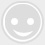# 干货丨100组雅思听力阅读必备同义词替换

-回复 -浏览8-10月写作口语预测讲解，获取视频>>

1. ignore=pay no attention=not pay any attention=take no notice=not take any notice 忽略，无视 v.

2. encounter=face=confront=meet 遇见，遭遇 v.

3. mistaken view=misconception 错误的观点 n.

4. easy to reach=accessible 容易达到的 adj.

5. change=modify(modification) 改变 v.

6. destruction=destroy=loss 毁坏 n.

7. investigate=make inquiries=enquiries=go into=probe=look into 调查 v.

10. reason=cause=factor=origins=root=stimulus

=impetus 原因 n.

11. protect=guard=safeguard=shield=conservation

12. newspapers, television=media 媒体 n.

13. where to live=habitat 居住地 n.

14. get warmer=global warming 变暖 n.

15. contribute to=play a part 有助于 v.

16. survive=continuing existence 幸存，活下来 v.

17. curriculum=course=syllabus 课程 n.

19. underdeveloped=degenerated=rudimentary

=no good at something=weak 贫穷的，可怜的 adj.

21. exceptional=good=excellent=outstanding

22. mating=courtship 交配 n.

24. good vision ability=vision is obviously more useful… 好的视力

25. best=well=greatest=finest=ideal=top=number one =ultimate=optimum=definitive=unsurpassed=record-breaking 极好的 adj.

26. surprised=amazed=astonished=astounded=be taken aback=startled 感到惊讶的 adj.

27. volunteer=subject 实验研究对象 n.

29. blind=can not see 瞎的 adj.

31. increase=go up=rise=grow=climb=gain

=escalate=pick up=widen=be on the increase=intensify=expand=build up 上升，增加 v.

32. teach=educate=train=coach=instruct=bring up

33. more than one language=bilingual

=sb’s second language 多种语言 n.

34. determine=establish=identify=pinpoint=diagnose 决定 v.

36. community=all the people in a particular area, city, country 群体，团体，社区 n.

38. fail=failure=not succeed=unsuccessful=vain=fruitless

=unproductive=be a failure=go wrong=not work

=do no good=fall through 失败 adj.

39. endanger=to put someone or something in danger of being hurt=dameged=destroyed 危及，使危险 v.

41. inevitable=certain to happen and impossible to avoid 不可避免的 adj.

42. differ from=unusual 与…不同 v.

45. alternative=another=other=different=new

46. therapist=someone who has been trained to give a particular form of treatment for physical or mental illness 临床医学家 n.

47. retrain=taking courses 再教育 v.

48. salary=pay=wage=income=earnings=fee 工资，收入 n.

49. long-term=chronic=long-running=long-standing

=lasting=lifelong=prolonged=lingering=enduring

50. complaint=complain=make a complaint=protest=object=complain 抱怨 n.

51. illness=disease=virus=bug=infection 疾病 n.

53. beneficial=be good for you=do somebody good=wholesome=nutritious=nourishing=healthful 有益的 adj.

54. insight=comprehension=understanding 理解，见解 n.

55. physical=relating to the body=bodily 身体的 adj.

56. hazard=risk=danger=threat 障碍，危险 n.

57. accompany=with=together=along with=come with 陪伴,伴随 v.

58. mental=relating to the health or state of someone's mind 精神上的，心理的 adj.

59. possible=possibility=can=potential=there is a chance/possibility 可能的 adj.

60. reduction=decrease=drop=fall=cut 减少 n.

61. class=type=kind=sort=style=category=variety 种类 n.

62. rehearsal=practice=training=run-through=exercise 排练，演习 n.

63. peer=your peers are the people who are the same age as you, or who have the same type of job, social class etc. 同伴 n.

64. defensive=used or intended to protect someone or something against attack 防御 n.

65. strategy=way=method=approach

=technique=system=tactics 方法，功略 n.

66. assist=help=aid=with the aid of 帮助 v.

67. specific=give (somebody) more details=expand on=enlarge on=go into more=greater detail=be more specific=be more explicit=elaborate 特定的 adj.

68. substance=a particular type of solid, liquid, or gas 物质 n.

69. surroundings=environment=circumstance 环境 n.

71. combine=mix=stir=beat=blend=whisk=dilute 联合 v.

72. survival=the state of continuing to live or exist 幸存 n.

73. quotation=a sentence or phrase from a book, speech etc. which you repeat in a speech or piece of writing because it is interesting or amusing 引言 n.

74. exemplify=example=case=instance=to be a very typical example of something=to give an example of something 例证 v.

75. explain=tell=say what/why/where etc.=show=demonstrate=go through=throw/shed light on=set out 解释 v.

76. outline=to describe something in a general way, giving the main points but not the details 概述 v.

77. purpose=aim=point=idea=objective

=object=goal=target=end 目的 n.

78. encourage=persuade=persuasion=get somebody to do something=influence=encourage=talk somebody into=put somebody up to 鼓励 v.

79. loan=an amount of money that you borrow from a bank etc. 贷款 n.

80. poverty=being poor 贫穷 n.

81. crime=illegal activities in general 犯罪 n.

82. reject=to refuse to accept, believe in, or agree with something 拒绝 v.

84. courier=a person or company that is paid to take packages somewhere 快递员 n.

85. storage=keep=store=keep something in storage=preserve 存储 n.

86. facility=rooms, equipment, or services that are provided for a particular purpose 设备 n.

88. effort=to try very hard to do something=attempt=campaign=drive 努力 n.

89. feature=a part of something that you notice because it seems important, interesting, or typical; a part of the land, especially a part that you can see; a part of someone's face, such as their eyes, nose etc. 特点 n.

90. planet=the world=earth=the globe 地球 n.

91. unpredictable=can't say/tell=unforeseeable=it remains to be seen 无法预测的 adj.

92. machinery=machines, especially large ones=a system or set of processes for doing something=machine=device=robot=appliance=mechanism 机械 n.

93. ultimate=someone's ultimate aim is their main and most important aim, that they hope to achieve in the future=final 最终的，根本的 adj.

94. atmosphere=the feeling that an event or place gives you=the mixture of gases that surrounds the Earth 气氛; 大气 n.

95. occur=happen=take place=there is=come about=come up=turn up=arise=strike 发生 v.

96. expand=get bigger=grow=swell up=stretch 扩张，扩大 v.

97. form=type=way something is/appears=shape 形式 n.

98. emerge=appear=become visible=come into view/come into sight=come out=reappear 出现 v.

99. emit=to send out gas, heat, light, sound etc. 发出，放射 n.

100. situation=circumstances=position=case=plight 情况，状况 n.